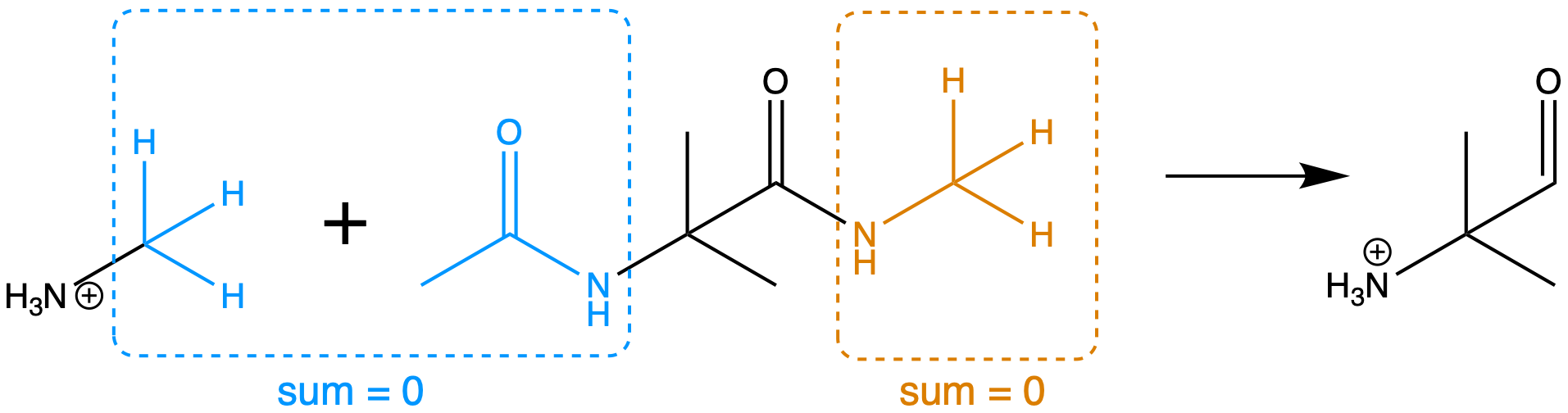# Calculating charges of multiple molecules with a temporary server

In this tutorial, we calculate the RESP charges of two molecules with a temporary server, incorporating intra-molecular and inter-molecular charge constraints. This is particularly handy for setting charge constraints between molecules, e.g. constraining groups of atoms in both molecules to sum to 0.

It is highly recommended to go over “Calculating charges of one molecule with a temporary server” first, as it explores some basic concepts of RESP more deeply.

:

import psiresp


## Creating the molecule

When we set up these molecules, let’s turn off geometry optimization to save some time.

:

nme2ala2 = psiresp.Molecule.from_smiles("CC(=O)NC(C)(C)C(NC)=O",
optimize_geometry=False)
nme2ala2.qcmol

:

methylammonium = psiresp.Molecule.from_smiles("C[NH3+]",
optimize_geometry=False)
methylammonium.qcmol


Let’s set up some charge constraints. We can do this with SMILES strings as well, to minimise confusion with indices. SMILES matches can be created either with mapped atoms (with numbers), or without. When only some atoms are mapped, only the numbered atoms are returned from the match, and in order of the numbers.

We will create constraints to match the scheme displayed below.For example, the below code selects the orange group -NH-CH3.

:

constraints = psiresp.ChargeConstraintOptions()
nme_smiles = "CC(=O)NC(C)(C)C([N:1]([H:2])[C:3]([H:4])([H:5])([H:6]))=O"
nme_indices = nme2ala2.get_smarts_matches(nme_smiles)
print(nme_indices)

[(8, 21, 9, 22, 23, 24)]


Below we use these indices to add a ChargeSumConstraint; the charges of all these atoms must sum to 0.

:

constraints.add_charge_sum_constraint_for_molecule(nme2ala2,
charge=0,
indices=nme_indices)
print(constraints.charge_sum_constraints)

[ChargeSumConstraint(atoms={Atom(index=22, molecule=Molecule(name=C7H14N2O2, charge=0) with 0 conformers), Atom(index=9, molecule=Molecule(name=C7H14N2O2, charge=0) with 0 conformers), Atom(index=24, molecule=Molecule(name=C7H14N2O2, charge=0) with 0 conformers), Atom(index=8, molecule=Molecule(name=C7H14N2O2, charge=0) with 0 conformers), Atom(index=21, molecule=Molecule(name=C7H14N2O2, charge=0) with 0 conformers), Atom(index=23, molecule=Molecule(name=C7H14N2O2, charge=0) with 0 conformers)}, charge=0.0)]


When no atoms are given map numbers, the whole match is returned. This constraint specified is the blue group shown in the image above.

:

methyl_atoms = methylammonium.get_atoms_from_smarts("C([H])([H])([H])")
ace_atoms = nme2ala2.get_atoms_from_smarts("C([H])([H])([H])C(=O)N([H])")
constraint_atoms = methyl_atoms + ace_atoms
constraints.charge_sum_constraints[-1]

:

ChargeSumConstraint(atoms={Atom(index=3, molecule=Molecule(name=C7H14N2O2, charge=0) with 0 conformers), Atom(index=12, molecule=Molecule(name=C7H14N2O2, charge=0) with 0 conformers), Atom(index=4, molecule=Molecule(name=CH6N, charge=1) with 0 conformers), Atom(index=2, molecule=Molecule(name=C7H14N2O2, charge=0) with 0 conformers), Atom(index=3, molecule=Molecule(name=CH6N, charge=1) with 0 conformers), Atom(index=11, molecule=Molecule(name=C7H14N2O2, charge=0) with 0 conformers), Atom(index=0, molecule=Molecule(name=CH6N, charge=1) with 0 conformers), Atom(index=1, molecule=Molecule(name=C7H14N2O2, charge=0) with 0 conformers), Atom(index=14, molecule=Molecule(name=C7H14N2O2, charge=0) with 0 conformers), Atom(index=0, molecule=Molecule(name=C7H14N2O2, charge=0) with 0 conformers), Atom(index=13, molecule=Molecule(name=C7H14N2O2, charge=0) with 0 conformers), Atom(index=2, molecule=Molecule(name=CH6N, charge=1) with 0 conformers)}, charge=0.0)


We can also constrain atoms to have equivalent charges. For example, the below constrains the hydrogens of the two middle methyls to all have the same charge.

:

h_smiles = "C(C([H:2])([H:2])([H:2]))(C([H:2])([H:2])([H:2]))"
h_atoms = nme2ala2.get_atoms_from_smarts(h_smiles)
print(constraints.charge_equivalence_constraints[-1])

atoms={Atom(index=16, molecule=Molecule(name=C7H14N2O2, charge=0) with 0 conformers), Atom(index=15, molecule=Molecule(name=C7H14N2O2, charge=0) with 0 conformers), Atom(index=18, molecule=Molecule(name=C7H14N2O2, charge=0) with 0 conformers), Atom(index=17, molecule=Molecule(name=C7H14N2O2, charge=0) with 0 conformers), Atom(index=20, molecule=Molecule(name=C7H14N2O2, charge=0) with 0 conformers), Atom(index=19, molecule=Molecule(name=C7H14N2O2, charge=0) with 0 conformers)}


## Setting up a server

Again, we will use QCFractal’s FractalSnowflakeHandler as a server.

:

from qcfractal import FractalSnowflakeHandler

server = FractalSnowflakeHandler()
print(server)

FractalSnowflakeHandler(name='db_caf859ff_7803_4bef_b986_3086b22cc865' uri='https://localhost:55263')

:

client = server.client()
print(client)

FractalClient(server_name='FractalSnowFlake_db_caf85', address='https://localhost:55263/', username='None')


## Running the job

The typical method and basis set for QM computation are “hg/6-31g*“, but we go with”b3lyp/sto-3g” here to save time.

:

geometry_options = psiresp.QMGeometryOptimizationOptions(
method="b3lyp", basis="sto-3g")
esp_options = psiresp.QMEnergyOptions(
method="b3lyp", basis="sto-3g",
)


To set up the job, we need to pass in the molecules (in any order), charge constraints, and QM options.

:

job_multi = psiresp.Job(molecules=[methylammonium, nme2ala2],
charge_constraints=constraints,
qm_optimization_options=geometry_options,
qm_esp_options=esp_options,)


Now we can run with the client.

:

job_multi.run(client=client)

generate-conformers: 100%|████████████████████████████████████████████████████████████████████████████████████████| 2/2 [00:00<00:00, 177.09it/s]
compute-esp: 100%|█████████████████████████████████████████████████████████████████████████████████████████████████| 2/2 [00:00<00:00, 10.83it/s]

:

[array([-0.1467648457182283, -0.0735202657457412,  0.1196505170703342,
0.1196505170703342,  0.1196505170703342,  0.2868916370108453,
0.2874443476006645,  0.2869975756414569]),
array([-0.2774379875879986,  0.449171254142842 , -0.3582897679346354,
-0.4879486568171876,  0.2498749830260423, -0.214333659946615 ,
-0.2111860805383453,  0.4065468062382359, -0.3658225841269276,
-0.2730302565730673, -0.3238946958042974,  0.0766343778467062,
0.0766343778467063,  0.0766343778467063,  0.2324153191640865,
0.0508632254196257,  0.0508632254196257,  0.0508632254196256,
0.0508632254196254,  0.0508632254196257,  0.0508632254196257,
0.2996953599909778,  0.1130524935696724,  0.1130524935696724,
0.1130524935696723])]


The charges are simply given as a list of charges, in the order of the molecules:

:

print(job_multi.charges)
print(job_multi.molecules.to_smiles())
print(job_multi.charges)
print(job_multi.molecules.to_smiles())

[-0.1467648457182283 -0.0735202657457412  0.1196505170703342
0.1196505170703342  0.1196505170703342  0.2868916370108453
0.2874443476006645  0.2869975756414569]
[C:1](-[N+:2](-[H:6])(-[H:7])-[H:8])(-[H:3])(-[H:4])-[H:5]
[-0.2774379875879986  0.449171254142842  -0.3582897679346354
-0.4879486568171876  0.2498749830260423 -0.214333659946615
-0.2111860805383453  0.4065468062382359 -0.3658225841269276
-0.2730302565730673 -0.3238946958042974  0.0766343778467062
0.0766343778467063  0.0766343778467063  0.2324153191640865
0.0508632254196257  0.0508632254196257  0.0508632254196256
0.0508632254196254  0.0508632254196257  0.0508632254196257
0.2996953599909778  0.1130524935696724  0.1130524935696724
0.1130524935696723]
[C:1](-[C:2](=[O:3])-[N:4](-[C:5](-[C:6](-[H:16])(-[H:17])-[H:18])(-[C:7](-[H:19])(-[H:20])-[H:21])-[C:8](-[N:9](-[C:10](-[H:23])(-[H:24])-[H:25])-[H:22])=[O:11])-[H:15])(-[H:12])(-[H:13])-[H:14]


Alternatively, you can inspect the actual charge attributes of each molecule. This can be useful for looking at the stage 1 vs stage 2, or restrained vs unrestrained, results.

:

print(job_multi.molecules.stage_2_restrained_charges)

[-0.1467648457182283 -0.0735202657457412  0.1196505170703342
0.1196505170703342  0.1196505170703342  0.2868916370108453
0.2874443476006645  0.2869975756414569]